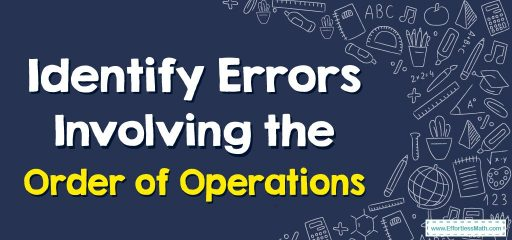# How to Identify Errors Involving the Order of Operations?

When an expression has two or more mathematical operations, you need to follow the order of operation rules. You can learn these rules in this post.When an expression has multiple operations, you have to follow this order of operation:

• First of all, do the operations inside the parentheses and the brackets.
• Then, calculate the exponents.
• After that, multiply and divide from left to right.
• And finally, add and subtract from left to right.

Errors involving the order of operations can be identified by using the acronym PEMDAS, which stands for Parentheses, Exponents, Multiplication, and Division (from left to right), and Addition and Subtraction (from left to right). Make sure to perform the operations in the correct order and to use parentheses as needed to specify the intended order of operations.

### Identifying Errors Involving the Order of Operations – Examples 1

Which operation is to be done first? Calculate.
$$18÷2+8$$
Solution:
First, you have to do the division. $$18÷2=9$$
Then add. $$9+8=17$$

### Identifying Errors Involving the Order of Operations – Examples 2

Which operation is to be done first? Calculate.
$$42÷\frac{6×5}{1+4}$$
Solution:
First, do the multiplication. $$6×5=30$$
Then, do the addition. $$1+4=5$$
Then divide $$30$$ by $$5$$. $$30÷5=6$$
And finally, divide $$42$$ by $$6$$. $$42÷6=8$$

### What people say about "How to Identify Errors Involving the Order of Operations? - Effortless Math: We Help Students Learn to LOVE Mathematics"?

No one replied yet.

X
30% OFF

Limited time only!

Save Over 30%

SAVE $5 It was$16.99 now it is \$11.99